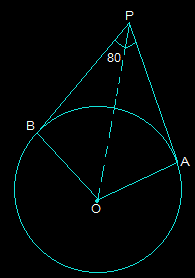Guru

# If tangents PA and PB from a point P to a circle with centre O are inclined to each other at angle of 80°, then ∠ POA is equal to (A) 50° (B) 60° (C) 70° (D) 80° Q.3

• 0

Sir give me the easy way to solve this problem from exercise 10.2 of class 10th of circles chapter of question no.3, this is an important question for class 10th give me the easy and simple way If tangents PA and PB from a point P to a circle with centre O are inclined to each other at angle of 80°, then ∠ POA is equal to (A) 50° (B) 60° (C) 70° (D) 80°

Share

1. First, draw the diagram according to the given statement.Now, in the above diagram, OA is the radius to tangent PA and OB is the radius to tangent PB.

So, OA is perpendicular to PA and OB is perpendicular to PB i.e. OA ⊥ PA and OB ⊥ PB

So, ∠OBP = ∠OAP = 90°

Now, in the quadrilateral AOBP,

The sum of all the interior angles will be 360°

So, ∠AOB+∠OAP+∠OBP+∠APB = 360°

Putting their values, we get,

∠AOB + 260° = 360°

∠AOB = 100°

Now, consider the triangles △OPB and △OPA. Here,

AP = BP (Since the tangents from a point are always equal)

OA = OB (Which are the radii of the circle)

OP = OP (It is the common side)

Now, we can say that triangles OPB and OPA are similar using SSS congruency.

∴△OPB ≅ △OPA

So, ∠POB = ∠POA

∠AOB = ∠POA+∠POB

2 (∠POA) = ∠AOB

By putting the respective values, we get,

=>∠POA = 100°/2 = 50°

As angle ∠POA is 50° option A is the correct option.

• 0